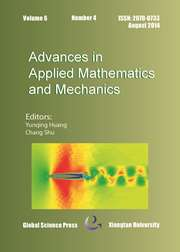Home
Hostname: page-component-99c86f546-n7x5d Total loading time: 0.344 Render date: 2021-12-02T20:32:28.919Z Has data issue: true Feature Flags: { "shouldUseShareProductTool": true, "shouldUseHypothesis": true, "isUnsiloEnabled": true, "metricsAbstractViews": false, "figures": true, "newCiteModal": false, "newCitedByModal": true, "newEcommerce": true, "newUsageEvents": true }Advances in Applied Mathematics and Mechanics

# Generating Function Methods for Coefficient-Varying Generalized Hamiltonian Systems

Published online by Cambridge University Press:  03 June 2015

*
*Corresponding author. Email: lixy1217@163.com

## Abstract

The generating function methods have been applied successfully to generalized Hamiltonian systems with constant or invertible Poisson-structure matrices. In this paper, we extend these results and present the generating function methods preserving the Poisson structures for generalized Hamiltonian systems with general variable Poisson-structure matrices. In particular, some obtained Poisson schemes are applied efficiently to some dynamical systems which can be written into generalized Hamiltonian systems (such as generalized Lotka-Volterra systems, Robbins equations and so on).

Type
Research Article
Information
Advances in Applied Mathematics and Mechanics , February 2014 , pp. 87 - 106

## Access options

Get access to the full version of this content by using one of the access options below. (Log in options will check for institutional or personal access. Content may require purchase if you do not have access.)

## References

Austin, M., Krishnaprasad, P. S. and Wang, L. S., Almost poisson integration of rigid body systems, J. Comput. Phys., 107 (1993), pp. 105117.CrossRefGoogle Scholar
Chen, C. M., Tang, Q. and Hu, S. F., Finite element method with superconvergence for nonlinear Hamiltonian systems, J. Comput. Math., 29 (2011), pp. 167184.Google Scholar
Cohen, D. and Hairer, E., Linear energy-preserving integrators for Poisson systems, BIT Numer. Math., 51 (2011), pp. 91101.CrossRefGoogle Scholar
Ergenc, T. and Karasozen, B., Poisson integrators for Volterra lattice equations, Appl. Numer. Math., 56 (2005), pp. 870887.Google Scholar
Feng, K. and Qin, M. Z., Symplectic Geometric Algorithms for Hamiltonian Systems, Springer-Verlag, 2010.CrossRefGoogle Scholar
Feng, K. and Wang, D. L., Symplectic difference schemes for Hamiltonian systems in general symplectic structures, J. Comput. Math., 9 (1991), pp. 8696.Google Scholar
Feng, K., Wu, H. M. and Qin, M. Z., Symplectic difference schemes for the linear Hamiltonian canonical systems, J. Comput. Math., 8 (1990), pp. 371380.Google Scholar
Feng, K., Wu, H. M., Qin, M. Z. and Wang, D. L., Construction of canonical difference schemes for Hamiltonian formalism via generating functions, J. Comput. Math., 7 (1989), pp. 7196.Google Scholar
Hairer, E., Lubich, C. and Wanner, G., Geometric Numerical Integration, Structure-Preserving Algorithms for Ordinary Differential Equations, Springer-Verlag, 2002.Google Scholar
HernáNdez-Bermejo, B. and FairéN, V., Hamiltonian structure and Darboux Theorem for families of generalized Lotka-Volterra systems, J. Math. Phys., 39 (1998), pp. 61626174.CrossRefGoogle Scholar
Hong, J. L. and Sun, Y. J., Generating functions of multi-symplectic RK methods via DW Hamilton-Jacobi equations, Numer. Math., 110 (2008), pp. 491519.CrossRefGoogle Scholar
Jay, L. O., Preserving Poisson structure and orthogonality in numerical integration of differential equations, Computers Math. Appl., 48 (2004), pp. 237255.CrossRefGoogle Scholar
Li, J. B., Zhao, X. H. and Liu, Z. R., Theory and Application of Generalized Hamiltonian Systems, Science Press, 1994.Google Scholar
Liu, X. S., Qi, Y. Y., He, J. F. and Ding, P. Z., Recent progress in symplectic algorithms for use in quantum systems, Commun. Comput. Phys., 2 (2007), pp. 153.Google Scholar
Makazaga, J. and Murua, A., A new class ofsymplectic integration schemes based on generating functions, Numer. Math., 113 (2009), pp. 631642.CrossRefGoogle Scholar
Marsden, J. E. AND Ratiu, S. T., Introduction to Mechanics and Symmetry, SpringerVerlag, 1994.CrossRefGoogle Scholar
Olver, P. J., Applications of Lie Groups to Differential Equations, Springer-Verlag, 1993.CrossRefGoogle Scholar
Tang, T. and Xu, X., Accuracy enhancement using spectral postprocessing for differential equations and integral equations, Commun. Comput. Phys., 5 (2009), pp. 779792.Google Scholar
Wang, D. L., Poisson difference schemes for Hamiltonian systems on Poisson manifolds, J. Comput. Math., 9 (1991), pp. 115124.Google Scholar
Zhang, S. Y. AND Deng, Z. C., Geometric Integration Theory and Application of Nonlinear Dynamical Systems, Northwestern Polytechnical University Press, 2005.Google Scholar

# Send article to Kindle

Note you can select to send to either the @free.kindle.com or @kindle.com variations. ‘@free.kindle.com’ emails are free but can only be sent to your device when it is connected to wi-fi. ‘@kindle.com’ emails can be delivered even when you are not connected to wi-fi, but note that service fees apply.

Find out more about the Kindle Personal Document Service.

Generating Function Methods for Coefficient-Varying Generalized Hamiltonian Systems
Available formats
×

# Send article to Dropbox

To send this article to your Dropbox account, please select one or more formats and confirm that you agree to abide by our usage policies. If this is the first time you use this feature, you will be asked to authorise Cambridge Core to connect with your <service> account. Find out more about sending content to Dropbox.

Generating Function Methods for Coefficient-Varying Generalized Hamiltonian Systems
Available formats
×

# Send article to Google Drive

To send this article to your Google Drive account, please select one or more formats and confirm that you agree to abide by our usage policies. If this is the first time you use this feature, you will be asked to authorise Cambridge Core to connect with your <service> account. Find out more about sending content to Google Drive.

Generating Function Methods for Coefficient-Varying Generalized Hamiltonian Systems
Available formats
×
×